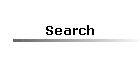### 3) Inline table-valued functions to return running jobs from msdb based on information from master..sysprocesses.

 fn_running_jobs.sql

### 5) Table-valued function that splits an Ascii string. Slightly different implementation of the above function.

 fn_c_split.sql

### 6) Scalar function that returns a string of bits for integer or binary values.

 fn_to_bitstr.sql

### 7) Inline table-valued function "BytesToBits" to get a range of bits from a 8-byte binary value.

 BytesToBits.sql

### 8) Scalar function "BitsToFloat" that returns sum of powers of 2 or inverse powers of 2 for a 8-byte IEEE 754 format binary value. The IEEE 754 format of the binary value can be obtained by doing cast( floatval as binary(8) ). This function is used by the utility functions that converts IEEE 754 format binary value to the floating point value. Note that SQL Server doesn't support supporting binary values to real/float directly.

 BitsToFloat.sql

### 9) Scalar functions to convert binary values of floating point numbers stored in internal format like in memory where the sequence of bytes are reversed. These functions convert the bytes into the IEEE 754 format with the bits numbered from left to right for convenience.

 BinaryToIEEE format.sql

### 10) Table-valued functions that return the binary ranges for single and double-precision floating point numbers. The binary values are in IEEE 754 format.

 IEEE 754 Tables.sql

### 11) Scalar function to convert 4-byte single-precision binary value in IEEE 754 format to real. The sp_ParseTrcShowStats stored procedure in the UtilitySPs page shows how this function is used to obtain real value from binary format.

 IEEE32ToReal.sql

### 12) Scalar function to convert 8-byte double-precision binary value in IEEE 754 format to float. The sp_ParseTrcShowStats stored procedure in the UtilitySPs page shows how this function is used to obtain float value from binary format.

 IEEE64ToFloat.sql